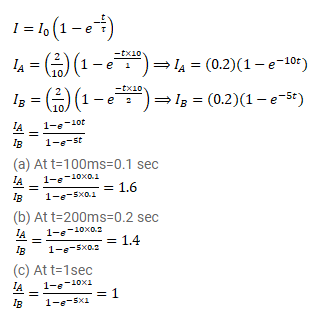# Two coils A and B have inductances 1.0H and 2.0H respectively.

Question:

Two coils $\mathrm{A}$ and $\mathrm{B}$ have inductances $1.0 \mathrm{H}$ and $2.0 \mathrm{H}$ respectively. The resistance of each coil is $10 \Omega$. Each coil is connected to an ideal battery of emf $2.0 \mathrm{~V}$ at $\mathrm{t}=0$. Let $i_{A}$ and $i_{B}$ be the currents in the two circuit at time t. Find the ratio $i_{A} / i_{B}$ at

(a) $\mathrm{t}=100 \mathrm{~ms}$,

(b) $\mathrm{t}=200 \mathrm{~ms}$ and

(c) $\mathrm{t}=1 \mathrm{~s}$.

Solution: